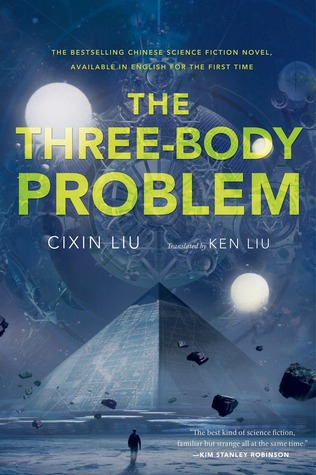# Book Review: The Three Body ProblemTitle:  The Three Body Problem

Author: Cixin Liu

Series? Yes, 1 of 3.

Rating: 4/5

I received this book from Harper Voyager Australia in exchange for an honest review.

One of the first things you’ll learn about me is that I detest physics and maths, I have absolutely no mental aptitude for it.  This is one of the first things you’ll see when you Google The Three Body Problem:${\ddot{\mathbf{x}}_\mathbf{1}} = -\frac{G m_2}{\left(x_1 - x_2\right)^3}\left(\mathbf{x_1} - \mathbf{x_2}\right)-\frac{G m_3}{\left(x_1 - x_3\right)^3}\left(\mathbf{x_1} - \mathbf{x_3}\right)$${\ddot{\mathbf{x}}_\mathbf{2}} = -\frac{G m_3}{\left(x_2 - x_3\right)^3}\left(\mathbf{x_2} - \mathbf{x_3}\right)-\frac{G m_1}{\left(x_2 - x_1\right)^3}\left(\mathbf{x_2} - \mathbf{x_1}\right)$${\ddot{\mathbf{x}}_\mathbf{3}} = -\frac{G m_1}{\left(x_3 - x_1\right)^3}\left(\mathbf{x_3} - \mathbf{x_1}\right)-\frac{G m_2}{\left(x_3 - x_2\right)^3}\left(\mathbf{x_3} - \mathbf{x_2}\right)$

I.e. DO. NOT. WANT.  However, I found that the book was surprisingly engaging and accessible despite its deep rooted background in theoretical physics.  Here’s why!NON-LINEAR PLOT:

The book follows two protagonists and timeline:

1.  Ye Wenjie, a physicist who watched her father persecuted and murdered during China’s Cultural Revolution during the 1970s, and
2. Wang Miao, a scientist researching nanofibre technology in present day.  He’s recruited by the police to investigate a group of scientists.  The reason?  Many scientists around the world have started committing suicide,

Ye’s story is the driving force of this book, watching her father’s execution for the crime of intelligence and progress has changed her fundamentally.  Her narratively examines humanity and the price we pay for scientific progress, it questions whether it’s all worth it.

“It was impossible to expect a moral awakening from humankind itself, just like it was impossible to expect humans to lift off the earth by pulling up on their own hair. To achieve moral awakening required a force outside the human race.”

Next to Ye, Wang is a slightly duller protagonist.  Through his eyes we are able to slowly uncover the rest of Ye’s story, as well as immerse in the titular Three-Body Problem.  Unfortunately, Wang is a bit of a blank slate, however, I did enjoy slowly seeing how he i) goes through the Three Body Game – I found myself cheering for him whenever he advanced in the game and ii) his story (and really, the story of the larger world) connects to Ye’s narrative. Continue reading “Book Review: The Three Body Problem”